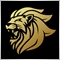# Cannot Open File - iCustom Error448

I am trying to return a value from an indicator buffer via iCustom, but I keep getting the error 'cannot open file':

I have declared the external variables in the correct order and everything appears to be written in the correct way:

`Value=iCustom(NULL,1440,"ADX & ADXR",C,B,0,1);`

But the error still appears.

I have posted the full code from the custom indicator and from the EA below.

The Custom Indicator:

```//+------------------------------------------------------------------+
//|                        Copyright 2018, MetaQuotes Software Corp. |
//|                                             https://www.mql5.com |
//+------------------------------------------------------------------+
#property version   "1.00"
#property strict
#property indicator_separate_window
#property indicator_buffers 5
#property indicator_color1 LightSkyBlue
#property indicator_color2 LightSalmon
#property indicator_color3 MediumSeaGreen
#property indicator_color4 Orange
#property indicator_color5 Gray
extern int Coefficient=14;
extern int Barrier_Level=25;
int i;
int j;
int Bar;
int Limit;
double Barrier[];
double plus24_TR;
double minus24_TR;
double TR_Take;
double plusDM;
double plusDM_Sum;
double plusDM_Take;
double plusDI[];
double minusDM;
double minusDM_Sum;
double minusDM_Take;
double minusDI[];
double True_Range;
double TR_Sum;
double DI_Difference;
double DI_Sum;
double DX;
double DX_Sum;
double DX_Count;
bool Mode;
//+------------------------------------------------------------------+
//| Custom indicator initialization function                         |
//+------------------------------------------------------------------+
int OnInit()
{
SetIndexBuffer(0, plusDI);
SetIndexBuffer(1, minusDI);
SetIndexBuffer(4,Barrier);
SetIndexStyle(0,DRAW_LINE,2,1,LightSkyBlue);
SetIndexStyle(1,DRAW_LINE,2,1,LightSalmon);
SetIndexStyle(2,DRAW_LINE,0,2,MediumSeaGreen);
SetIndexStyle(3,DRAW_LINE,0,2,Orange);
SetIndexStyle(4,DRAW_LINE,2,1,Gray);
SetIndexLabel(0,"+DI");
SetIndexLabel(1,"-DI");
SetIndexLabel(4,"Trend Barrier");
return(INIT_SUCCEEDED);
}
//+---------------------------------------------------------------------------------------------+
//| Custom indicator iteration function                                                         |
//+---------------------------------------------------------------------------------------------+
int OnCalculate(const int rates_total,
const int prev_calculated,
const datetime &time[],
const double &open[],
const double &high[],
const double &low[],
const double &close[],
const long &tick_volume[],
const long &volume[],
{
Limit=Bars-Coefficient;
int Counted_Bars=IndicatorCounted();
i=Bars-Counted_Bars-1;
if(i>0)
Mode=true;
if(i>Limit)
i=Limit;
Bar=i;

if(Mode==false)
{
plusDM_Sum = plusDM_Sum - plusDM;
minusDM_Sum = minusDM_Sum - minusDM;
TR_Sum = TR_Sum - True_Range;
}

while(i==Limit)
{
for(j=Limit; j<Bars-1; j++)
{
True_Range = TR_Function(Bar);

DM_Function(Bar,plusDM,minusDM);

TR_Sum        = TR_Sum      + True_Range;
plusDM_Sum    = plusDM_Sum  + plusDM;
minusDM_Sum   = minusDM_Sum + minusDM;
Bar++;
}

Barrier[i]=Barrier_Level;
i--;
Bar=i;
}

while(i<Limit && i>=0)
{
if(Mode==true)
{
plusDM_Sum   = Take_Function(plusDM_Sum,Coefficient);
minusDM_Sum  = Take_Function(minusDM_Sum,Coefficient);
TR_Sum       = Take_Function(TR_Sum,Coefficient);
}

True_Range   = TR_Function(Bar);

DM_Function(Bar,plusDM,minusDM);

TR_Sum       = TR_Sum      + True_Range;
plusDM_Sum   = plusDM_Sum  + plusDM;
minusDM_Sum  = minusDM_Sum + minusDM;

plusDI[i]     = plusDM_Sum  / TR_Sum * 100;
minusDI[i]    = minusDM_Sum / TR_Sum * 100;

DI_Difference = MathAbs(plusDI[i] - minusDI[i]);
DI_Sum        = plusDI[i] + minusDI[i];
DX            = DI_Difference / DI_Sum * 100;
DX_Count++;

if(DX_Count<Coefficient)
DX_Sum     = DX_Sum + DX;

if(DX_Count==Coefficient)
{
DX_Sum     = DX_Sum + DX;
}

if(DX_Count>Coefficient)

if(DX_Count>=Coefficient*2-1)

Barrier[i]=Barrier_Level;
i--;
Bar=i;

if(i<0)
Mode=false;
else
{
if(i>=0)
Mode=true;
}

}
return(rates_total);
}
//+------------------------------------------------------------------------------------------+
//| Take Function:                                                                           |
//+------------------------------------------------------------------------------------------+
double Take_Function(double Sum, int CO)
{
double Take = Sum / CO;
double New  = Sum - Take;
return(New);
}
//+------------------------------------------------------------------------------------------+
//| True Range Function:                                                                     |
//+------------------------------------------------------------------------------------------+
double TR_Function(int BAR)
{
double Range;
Range = MathAbs(High[BAR] - Low[BAR]);       // Intraday_TR
Range = MathAbs(High[BAR] - Close[BAR+1]);   // plus24_TR
Range = MathAbs(Low[BAR] - Close[BAR+1]);    // minus24_TR

int Max=ArrayMaximum(Range);
double New2=Range[Max];
return(New2);
}
//+------------------------------------------------------------------------------------------+
//| +DM & -DM Function:                                                                      |
//+------------------------------------------------------------------------------------------+
void DM_Function(int BAR, double& plus_DM, double& minus_DM)
{
//+-----------------------------------------------------------------+
//| standard +DM candle                                             |
//+-----------------------------------------------------------------+
if(High[BAR]>High[BAR+1] && Low[BAR]>=Low[BAR+1])
{
plusDM  = MathAbs(High[BAR] - High[BAR+1]);
minusDM = 0;
}
//+-----------------------------------------------------------------+
//| standard -DM candle                                             |
//+-----------------------------------------------------------------+
if(Low[BAR]<Low[BAR+1] && High[BAR]<=High[BAR+1])
{
minusDM = MathAbs(Low[BAR] - Low[BAR+1]);
plusDM  = 0;
}
//+-----------------------------------------------------------------+
//| outside day candle                                              |
//+-----------------------------------------------------------------+
if(High[BAR]>High[BAR+1] && Low[BAR]<Low[BAR+1])
{
double H2H = MathAbs (High[BAR] - High[BAR+1]);
double L2L = MathAbs (Low[BAR]  - Low[BAR+1]);
if(H2H>L2L) // plusDM outside day
{
plusDM  = H2H;
minusDM = 0;
}
else
{
if(L2L>H2H) // minusDM outside day
{
minusDM = L2L;
plusDM  = 0;
}
}
}
//+-----------------------------------------------------------------+
//| inside day candle                                               |
//+-----------------------------------------------------------------+
if(High[BAR]<=High[BAR+1] && Low[BAR]>=Low[BAR+1])
{
plusDM  = 0;
minusDM = 0;
}
}
//+------------------------------------------------------------------------------------------+ ```

The EA:

```#property copyright "Copyright 2018, MetaQuotes Software Corp."
#property version   "1.00"
#property strict
extern int Coefficient   = 14;
extern int Barrier_Level = 25;
double Value;
//+------------------------------------------------------------------+
//| Expert initialization function                                   |
//+------------------------------------------------------------------+
int OnInit()
{

return(INIT_SUCCEEDED);
}
//+------------------------------------------------------------------+
//| Expert tick function                                             |
//+------------------------------------------------------------------+
void OnTick()
{
int C = Coefficient;
int B = Barrier_Level;

}
//+------------------------------------------------------------------+
//| Expert deinitialization function                                 |
//+------------------------------------------------------------------+
void OnDeinit(const int reason)
{
}
//+------------------------------------------------------------------+```Moderator
12670

You should really rename it to something without spaces and non alphabetic characters.448

Marco vd Heijden:
You should really rename it to something without spaces and non alphabetic characters.

Thank you